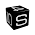## Wednesday, November 23, 2011

### How to make Bubble Charts with matplotlib

In this post we will see how to make a bubble chart using matplotlib. The snippet that we are going to see was inspired by a tutorial on flowingdata.com where R is used to make a bubble chart that represents some data extracted from a csv file about the crime rates of America by states. I used the dataset provided by flowingdata to create a similar chart with Python. Let's see the code:
```from pylab import *
from scipy import *

# reading the data from a csv file
durl = 'http://datasets.flowingdata.com/crimeRatesByState2005.csv'
rdata = genfromtxt(durl,dtype='S8,f,f,f,f,f,f,f,i',delimiter=',')

rdata = zeros(8) # cutting the label's titles
rdata = zeros(8) # cutting the global statistics

x = []
y = []
color = []
area = []

for data in rdata:
x.append(data) # murder
y.append(data) # burglary
color.append(data) # larceny_theft
area.append(sqrt(data)) # population
# plotting the first eigth letters of the state's name
text(data, data,
data,size=11,horizontalalignment='center')

# making the scatter plot
sct = scatter(x, y, c=color, s=area, linewidths=2, edgecolor='w')
sct.set_alpha(0.75)

axis([0,11,200,1280])
xlabel('Murders per 100,000 population')
ylabel('Burglaries per 100,000 population')
show()
```
The following figure is the resulting bubble chart It shows the number of burglaries versus the number of murders per 100,000 population. Every bubble is a state of America, the size of the bubbles represents the population of the state and the color is the number of larcenies.

1.Pretty cool! It is amazing what matplotlib does this days!

2.Neat.

Looking at your code for slicing up the CSV file, I think you might want to check out pandas, a package for handling tabular data like this: http://pandas.sourceforge.net/

3.if data is population, don't you want area.append(data) instead of the square root? It seems to me that each person in the population should represent a certain area and, therefore, the area of the circle would be proportional to the population, not sqrt(population).

4.I use the square root to scale the area.

5.How do you add a bubble plot legend in matplotlib where the Legend shows a representation of the bubble sizes... i.e. say 1million - 5 million - 10million - 15million bubble sizes

1.I believe there's no built way to that. You could use a subplot which shows some bubbles aligned with the number which is represented by the size of the bubble.

6.Thank you for this useful piece of code! Simple and clean.

If you learn of a nice way to deal with legends - please update!

7.At least as of matplotlib 1.3.1, the marker size for scatter plots is already scaled by area (see scatter plot documentation) Using the square root gives the incorrect marker sizes..

8.Thanks for this article. I was looking for a way to get started doing bubble charts using Python.

1.Hi Shawn. Another place to start - I know I am late with this comment - is with the seaborn.scatterplot(). This is a super powerful tool that can make vary size and color by categorial or numeric value. It was developed quite recently.

2.Yep. I've seen it. I'm still using Classic Python 2.5 though.